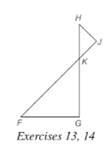Chapter 5.2, Problem 13E### Elementary Geometry for College St...

6th Edition
Daniel C. Alexander + 1 other
ISBN: 9781285195698

#### Solutions

Chapter
Section### Elementary Geometry for College St...

6th Edition
Daniel C. Alexander + 1 other
ISBN: 9781285195698
Textbook Problem
1 views

#If the drawing, △ H J K ∼ △ F G K . If H K = 6 , K F = 8 , and H J = 4 , find H J .

To determine

To calculate: The value of FG.

Explanation

Given:

ΔHJK~ΔFGK.

HK=6,KF=8 and HJ=4.

Approach:

Similarity between Two polygons:

Two polygons are similar if and only if when all pairs of corresponding angles are congruent and all pairs of corresponding sides are proportional.

Calculation:

According to the definition of similar polygon, FG¯HJ¯ and KF¯KH¯

So,

### Still sussing out bartleby?

Check out a sample textbook solution.

See a sample solution

#### The Solution to Your Study Problems

Bartleby provides explanations to thousands of textbook problems written by our experts, many with advanced degrees!

Get Started